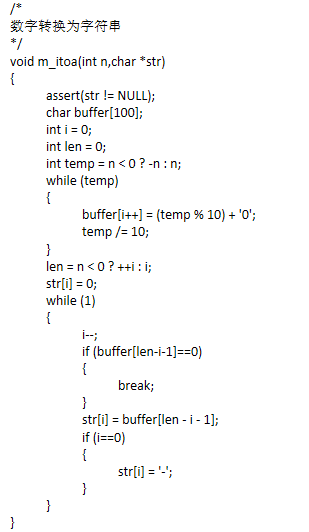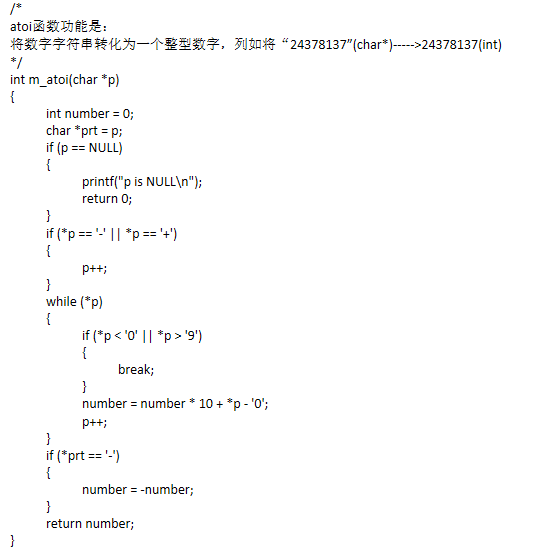• atoi库函数 #include <stdio.h> int main() { char*str = "4194";//字符串 int res = atoi(str);// 库函数 把一个字符转转换成一个整形 printf("res=%d\n", res); return 0; } atoi 是把一个字符串转换...
atoi库函数
#include <stdio.h>
int main()
{
char*str = "4194";//字符串
int res = atoi(str);// 库函数 把一个字符转转换成一个整形
printf("res=%d\n", res);
return 0;
}

atoi 是把一个字符串转换成一个整形
下面模拟实现一下 atoi
#include <stdio.h>
#include <assert.h>
#include <stdlib.h>
int my_atoi(const char*str)
{
assert(str != NULL);
//跳过空格  如果是 ‘  4194’这种情况
while (*str == ' ')// 跳过空格
{
str++;
}
int flag = 1;// 符号位
if (*str == '-')
{
flag = -1;  //如果有-  则置-1   看是否是负数
str++;  //str++ 判断后面的字符是否是数字
}

else if (*str == '+')
str++;  // str++ 判断后面的字符是否是数字
long long res = 0;
while (*str != '\0')
{
if (*str<'0' || *str>'9')// 如果是个字符 无效的字符串
break; //直接跳出
res = res * 10 + (*str - '0');//字符4是52-字符0就是真的数字4了   字符0是48
if (res > INT_MAX || -res < INT_MAX)  //判断这个数是否超出整形范围  若超范围了 返回0
return 0;
str++;
}
return flag*res;
}
int main()
{
char*str = "-4194";
int res1 = atoi(str);
printf("res1=%d\n", res1);
int res2 =my_atoi(str);
printf("res2=%d\n", res2);
return 0;
}



展开全文字符串
•展开全文• int atoi(const char *nptr); 用法：将字符串里的数字字符转化为整形数，返回整形值。 注意：转化时跳过前面的空格字符，直到遇上数字或正负符号才开始做转换，而再遇到非数字或字符串结束符号时('/0')才结束转换...
库函数原型：
#inclue <stdlib.h>
int atoi(const char *nptr);
用法：将字符串里的数字字符转化为整形数，返回整形值。
注意：转化时跳过前面的空格字符，直到遇上数字或正负符号才开始做转换，而再遇到非数字或字符串结束符号时('/0')才结束转换，并将结果返回。
例：
#include <stdio.h>#include <stdlib.h>
int main(){    char *ptr1 = "-12345.12";
char *ptr2 = "+1234w34";
char *ptr3 = "   456er12";
char *ptr4 = "789 123";
int a,b,c,d;
a = atoi(ptr1);    b = atoi(ptr2);    c = atoi(ptr3);
d = atoi(ptr4);
printf("a = %d, b = %d, c = %d, d = %d/n", a,b,c,d);
return 0;}
输出结果：a = 12345， b = 1234，
c = 456， d = 789
***************************************************
不调用库函数用C语言实现atoi函数的功能：
#include <stdio.h>#include <stdlib.h>
int my_atoi(const char *str);
int main(int argc, char *argv[]){    char *ptr = " 1234 3455";
int n;
n = my_atoi(ptr);    printf("myAtoi:%d/n", n);
n = atoi(ptr);    printf("atoi:%d/n", n);    return 0;
}
int my_atoi(const char *str){    int value = 0;
int flag = 1; //判断符号
while (*str == ' ')  //跳过字符串前面的空格    {        str++;
}
if (*str == '-')  //第一个字符若是‘-’，说明可能是负数    {        flag = 0;
str++;
}
else if (*str == '+') //第一个字符若是‘+’，说明可能是正数    {        flag = 1;
str++;
}//第一个字符若不是‘+’‘-’也不是数字字符，直接返回0    else if (*str >= '9' || *str <= '0')
{
return 0;
}
//当遇到非数字字符或遇到‘/0’时，结束转化    while (*str != '/0' && *str <= '9' && *str >= '0')    {
value = value * 10 + *str - '0'; //将数字字符转为对应的整形数        str++;    }
if (flag == 0) //负数的情况    {        value = -value;
}
return value;}


展开全文语言 c
• #include  #include    int main(void) {  float n;  char const *str = "12345.67";... n = atoi(str);  printf("string=%sint=%dfloat=%f\n",str,n,n);  return 0;

#include <stdlib.h>

#include <stdio.h>

int main(void)

{

    float n;

    char const *str = "12345.67";

    n = atoi(str);

    printf("string=%sint=%dfloat=%f\n",str,n,n);

    return
0;

}

输出：

string = 12345.67 int=12345float = 12345.000000


展开全文code
• https://www.cnblogs.com/ralap7/p/9171613.html
• ## Atoi函数C语言实现

千次阅读 2016-07-25 11:25:56
Atoi函数实现 这段时间在学习算法，发现以前学过的并且熟悉的知识，不用便很快忘记了，在leetcode上遇到了一个Atoi转换函数的实现，初看只是字符串转整型，但是实际上需要考虑很多边界情况。空格（tab、空格） atoi...leetcode c
• atoiatoi()函数是把字符串转化为数字。...a=atoi(str); /*将字符串转化为int型的数字*/printf("%d\n",a);}输出为123相关阅读定义和用法array_column() 返回输入数组中某个单一列的值。语法array_column(array,c...
• atoi 先来看看官方文档（传送门） 接口 int atoi (const char * str); Convert string to integer Parses the C-string str interpreting its content as an integral number, which is returned as a value of ...字符串 c++
• 现在关注微信公众号：C程序编程 免费获取进阶指导，资料工具以及源码程序设计要求用C语言写一个简单的火车售票系统，主要实现的功能为：录入班次信息浏览班次信息按班次号查询按终点站查询按余票数量排序保存售票...
• Implement atoi which converts a string to an integer.The function first discards as many whitespace characters as necessary until the first non-whitespace character is found. Then, starting from ...
• 请你来实现一个 myAtoi(string s) 函数，使其能将字符串转换成一个 32 位有符号整数（类似 C/C++ 中的 atoi 函数）。 函数 myAtoi(string s) 的算法如下： 读入字符串并丢弃无用的前导空格 检查下一个字符（假设还未...字符串
• 请你来实现一个 atoi 函数，使其能将字符串转换成整数。 首先，该函数会根据需要丢弃无用的开头空格字符，直到寻找到第一个非空格的字符为止。 当我们寻找到的第一个非空字符为正或者负号时，则将该符号与之后面尽...
• Implement atoi which converts a string to an integer. The function first discards as many whitespace characters as necessary until the first non-whitespace character is found. Then, starting from ...
• C语言实现atoi 文章目录C语言实现atoi前言一、实现思路二、代码示例 前言 请你来实现一个 myAtoi(string s) 函数，使其能将字符串转换成一个 32 位有符号整数（类似 C/C++ 中的 atoi 函数）。 函数 myAtoi(string ...字符串
• ## C语言atoi函数

千次阅读 2020-04-14 09:58:39
C语言提供了一系列函数把字符串转换为整数：atoi、atol、atoll和atoq。 1、包含头文件 #include <stdlib.h> 2、函数声明 int atoi(const char *nptr); long atol(const char *nptr); long long atoll(co...
• 请你来实现一个 myAtoi(string s) 函数，使其能将字符串转换成一个 32 位有符号整数（类似 C/C++ 中的 atoi 函数）。 函数 myAtoi(string s) 的算法如下： 读入字符串并丢弃无用的前导空格 检查第一个字符（假设还未...字符串
• （1）C语言itoa()函数和atoi()函数详解(整数转字符C实现) https://www.cnblogs.com/bluestorm/p/3168719.html...

# atoic语言c语言 订阅